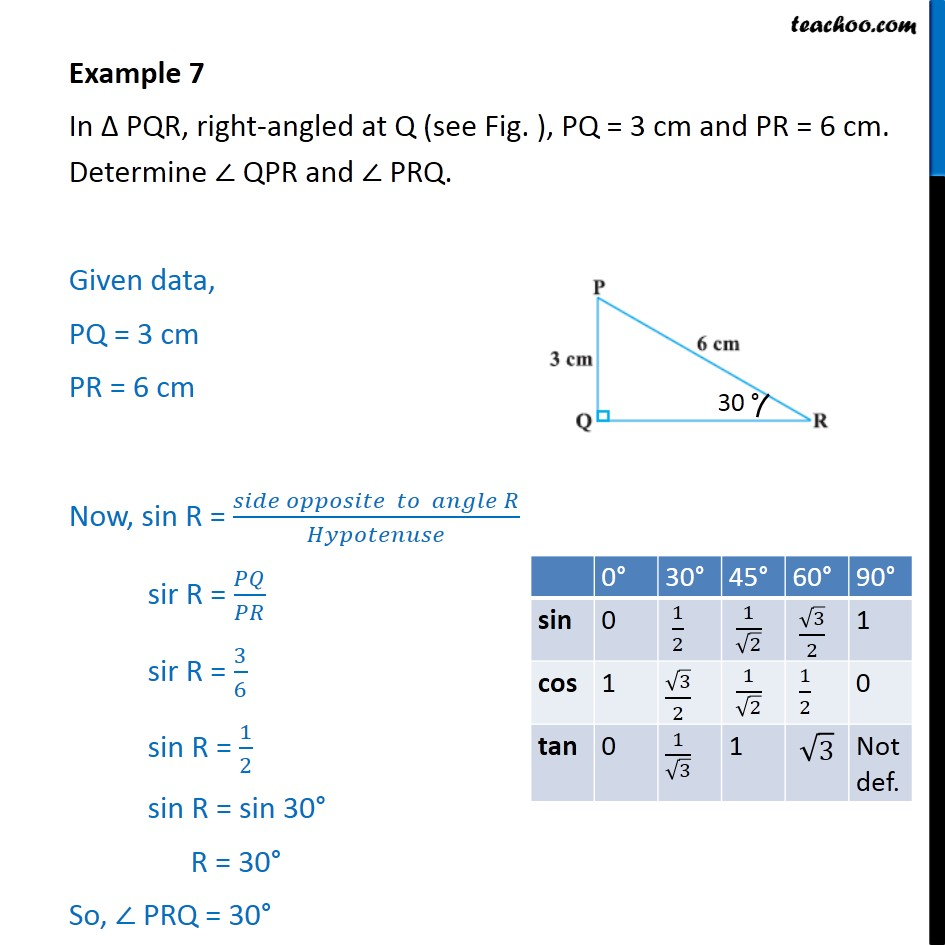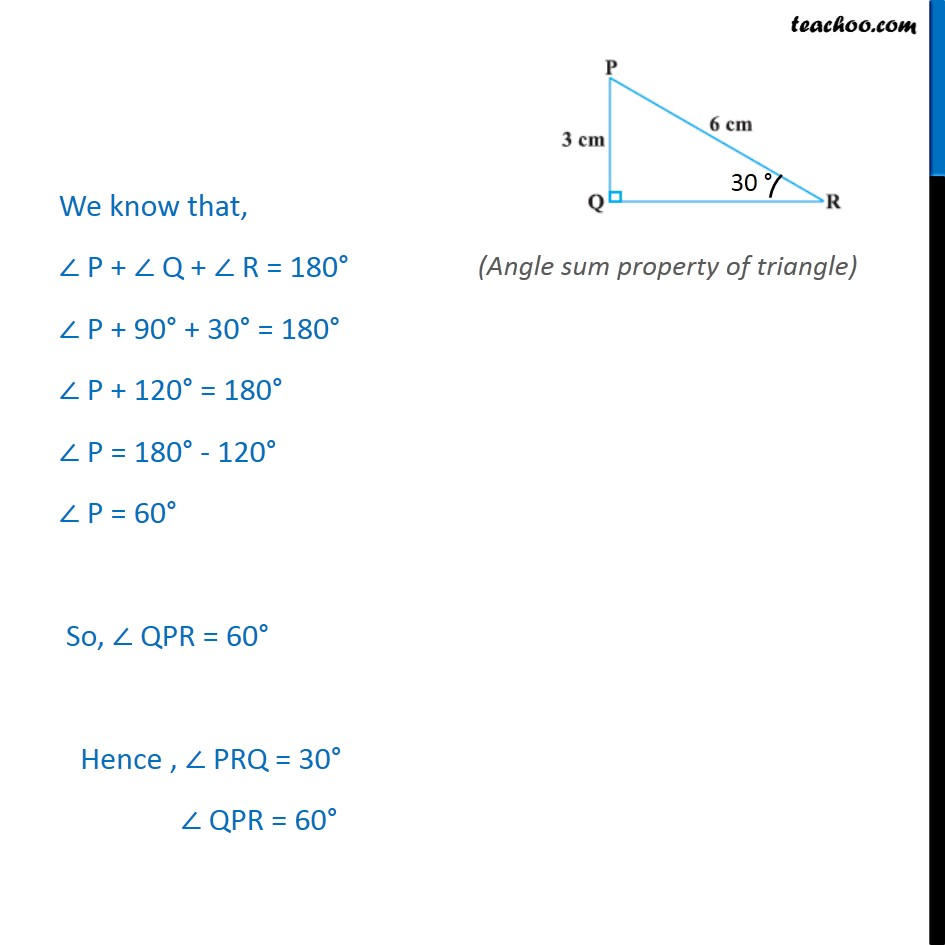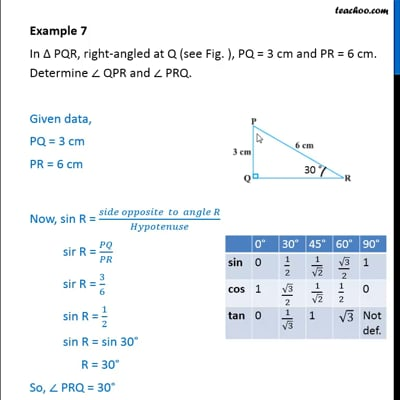Examples

Chapter 8 Class 10 Introduction to Trignometry
Serial order wiseThis video is only available for Teachoo black users

Solve all your doubts with Teachoo Black (new monthly pack available now!)

### Transcript

Example 7 In Δ PQR, right-angled at Q (see Fig. ), PQ = 3 cm and PR = 6 cm. Determine ∠ QPR and ∠ PRQ. Given data, PQ = 3 cm PR = 6 cm Now, sin R = (𝑠𝑖𝑑𝑒 𝑜𝑝𝑝𝑜𝑠𝑖𝑡𝑒 𝑡𝑜 𝑎𝑛𝑔𝑙𝑒 𝑅)/𝐻𝑦𝑝𝑜𝑡𝑒𝑛𝑢𝑠𝑒 sir R = 𝑃𝑄/𝑃𝑅 sir R = 3/6 sin R = 1/2 sin R = sin 30° R = 30° So, ∠ PRQ = 30° We know that, ∠ P + ∠ Q + ∠ R = 180° ∠ P + 90° + 30° = 180° ∠ P + 120° = 180° ∠ P = 180° - 120° ∠ P = 60° So, ∠ QPR = 60° Hence , ∠ PRQ = 30° ∠ QPR = 60°